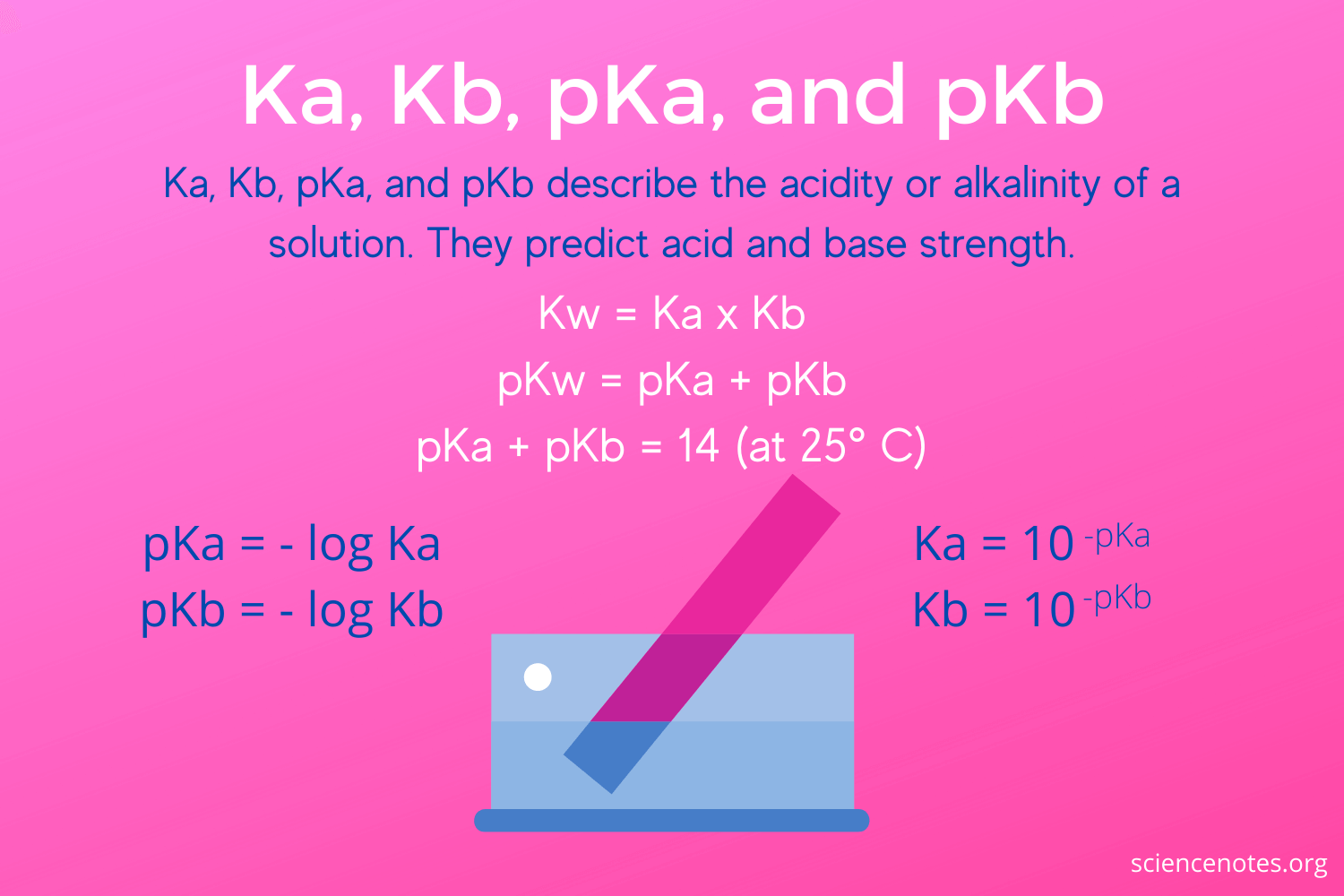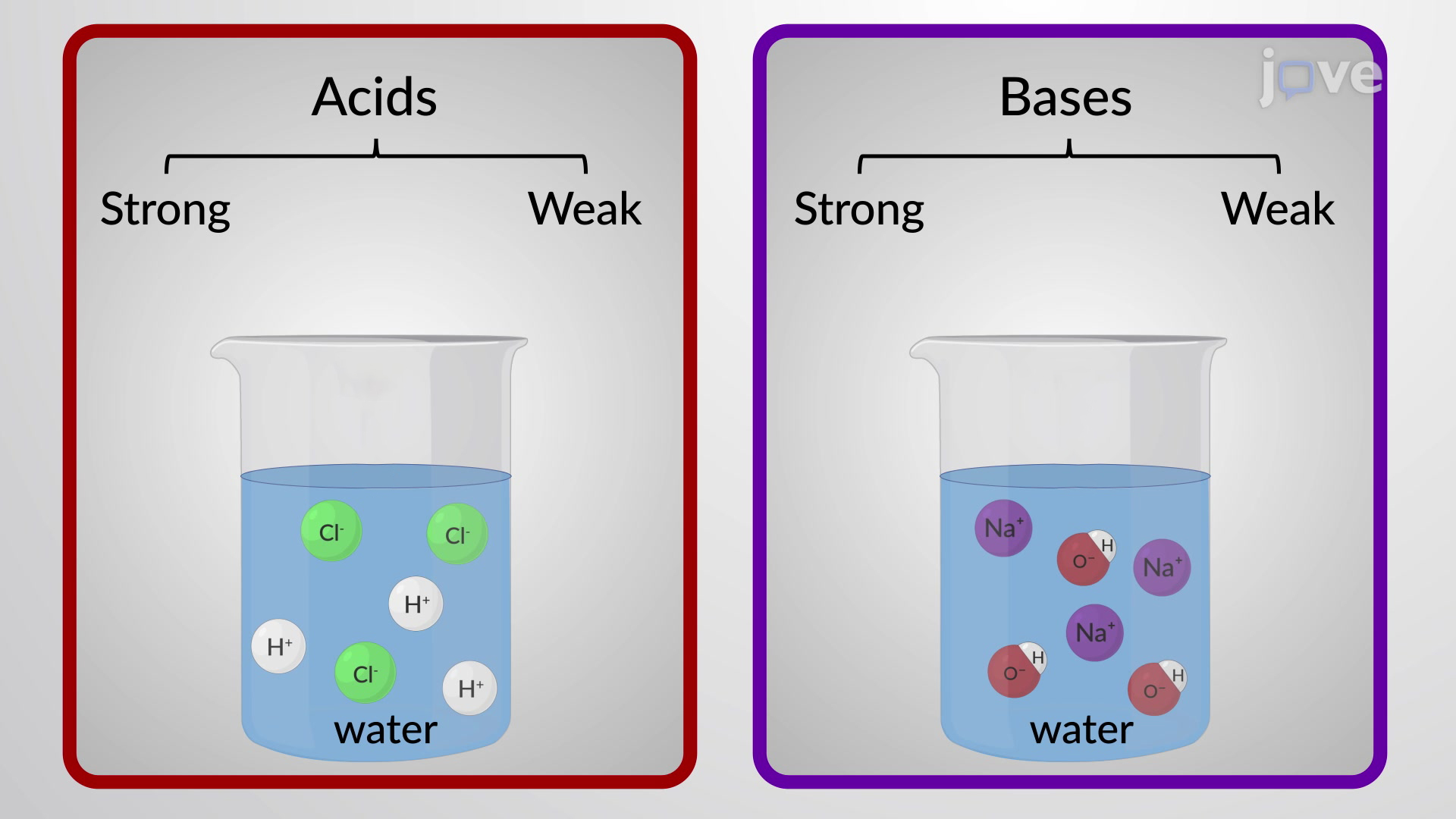Gayathri Mani

HS-PS1-2

# Calculating Ka and Kb Study Guide

The calculation of pKb and Kb is carried out to determine the strength of a base.

## INTRODUCTION

Cookies for a sweet tooth - Have you ever wondered why cookies 🍪 can be eaten even though they contain a strong base like baking soda while you have to be extremely careful with handling other bases, such as a drain cleaner 🤔? The answer is that some bases dissociate or break down very well while others do not.Source

### DISSOCIATION CONSTANTS

Dissociation constants are used to measure the way acids and bases dissociate or break down. The dissociation constant for acids is called Ka, and for bases is called Kb.

When a base is dissolved in water, there occurs an equilibrium reaction, and the base splits into B + H₂O ⇌ BH+ + OH-

Therefore, the equilibrium constant can be written as:

``````   Kb = [(BH+)(OH-) / B]
``````

The more the Kb value, the base will be stronger.

### WHAT IS PK IN CHEMISTRY?

The negative logarithm of Kb equilibrium or "p" can be written as pKb = - log Kb The lower the value of pKb, the stronger the base.

For instance, the Kb for a weak base such as NH3 can be calculated as

``````       NH₃ + H₂O = NH₄+ + OH-
``````

Therefore, Kb = [(NH₄+)(OH-)/NH₃]

``````      Kb (Ammonia) = 1.74 x 10-5
``````

For acids, when an acid dissociates in water,

HA + H₂O ⇌ H₃O+ + A-

So, Ka = [(H₃O+)(A-)/HA]

The higher the value of Ka, the acid is more powerful. Also, pKa = -log KaSource

### Ka Kb VALUES

• The Kb value for most bases ranges from 10⁻² to 10⁻¹³; i.e., pKb is 2 to 13.
• The Ka value for most bases ranges from 10⁻² to 10⁻¹⁴; i.e., pKa is 2 to 14.
• The ion constant for water is represented as Kw, which connects Kb and Ka as Kw = Kb x Ka
• Hence, the Ka Kb equation may be written as pKa + pKb = 14.

## CONCLUSION

• The strengths of acids and bases can be measured based on how they dissociate in water.
• The ion constant for water is connected to the equilibrium constants for acids and bases.

### FAQs:

1. How do you calculate Kb?

A. You can calculate K by dividing Kw by the value of Ka, which will be equal to the Kb constant or Kw = Kb x Ka

``````      So,  Kb = Kw / Ka
``````

2. What is the value of Kb?

A. The value of Kb ranges from 10⁻² to 10⁻¹³ for most bases.

3. What is Kb in chemistry?

Kb is the base dissociation constant and determines the extent to which a base dissociates into its respective ions in a medium like water.

4. What is the equation for KW?

The equation for water is written as Kw = Kb x Ka

We hope you enjoyed studying this lesson and learned something cool about the Calculating Kₐ and Kb! Join our Discord community to get any questions you may have answered and to engage with other students just like you! Don't forget to download our app to experience our fun VR classrooms - we promise it makes studying much more fun! 😎

## SOURCES:

1. Calculating Ka and Kb. https://www.ck12.org/c/chemistry/calculating-ka-and-kb/enrichment/Ka-Kb-pKa-and-pKb-Overview/. Accessed 18 February 2022.
2. pH, pKa, Ka, pKb, Kb. https://socratic.org/organic-chemistry-1/acids-and-bases-1/ph-pka-ka-pkb-kb. Accessed 18 Feb 2022.
3. Relationship between Ka, Kb, pKa, and pKb. https://chem.libretexts.org/Courses/Brevard_College/CHE_104%3A_Principles_of_Chemistry_II/07%3A_Acid_and_Base_Equilibria/7.12%3A_Relationship_between_Ka_Kb_pKa_and_pKb. Accessed 18 Feb 2022.
4. Calculating Ka and Kb. https://chem.libretexts.org/Courses/Brevard_College/CHE_104%3A_Principles_of_Chemistry_II/07%3A_Acid_and_Base_Equilibria/7.13%3A_Calculating_(K_texta)_and_(K_textb). Accessed 18 Feb 2022.